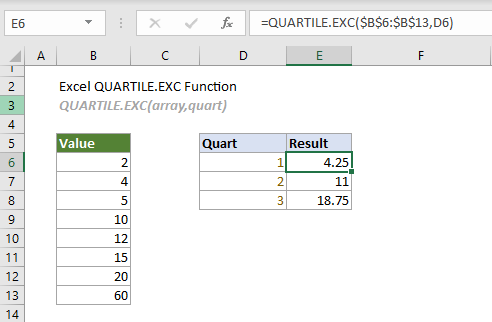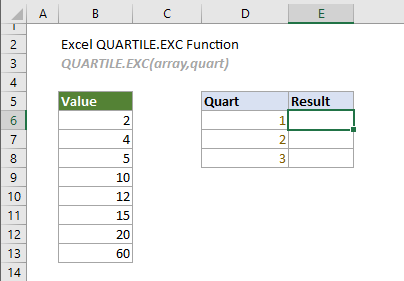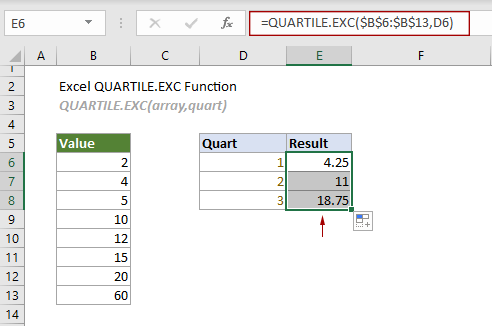## Excel QUARTILE.EXC function

The QUARTILE.EXC function returns the quartile for a given set of data based on a percentile range of 0 to 1 exclusive. It can be used to calculate the first quartile, second quartile and third quartile of a data set, but excludes the median when identifying Q1 and Q3.

This function is available in Excel 2010 and later versions.#### QUARTILE.EXC function VS. QUARTILE.INC function:

The difference between these two functions is that the QUARTILE.EXC function calculates the quartile of a data set based on a percentile range of 0 to 1 exclusive, whereas the QUARTILE.INC function calculates the quartile of a data set based on a percentile range of 0 to 1 inclusive.

#### Syntax

QUARTILE.EXC(array, quart)

#### Arguments

• Array (required): The array or cell range for which you want the quartile value;
• Quart (required): A number between 1 and 3 indicates which quartile value to return.
 Number Quartile value 1 The 1st quartile (25th percentile) 2 The 2nd quartile - median value (50th percentile) 3 The 3rd quartile (75th percentile)

#### Remarks

1. If “quart” is not an integer, it will be truncated;
2. The #NUM! error occurs when either of the following conditions is met;
-- “array” is empty;
-- “quart” < 0 or “quart” > 4.
3. The #VALUE! error occurs if “quart” is non-numeric;
4.If the requested quartile falls between two of the values in the “array”, Excel will interpolate between these values to calculate the quartile.

#### Return value

It returns a numeric value.

#### Example

Here is a list of values as shown in the screenshot below, to calculate the first quartile, second quartile and third quartile by using the QUARTILE.EXC function, you can do as follows.Select the top cell (E6 in this case), copy or enter the formula below and press Enter to get the result of the first quartile. Select this result cell and drag it AutoFill Handle down to get the second and third quartiles.

=QUARTILE.EXC(\$B\$6:\$B\$13,D6)Notes: The formula in cell E6 can be changed to:

=QUARTILE.EXC({2,4,5,10,12,15,20,60},0)

#### Related Functions

Excel QUARTILE.INC function
The QUARTILE.INC function returns the quartile for a given set of data based on a percentile range of 0 to 1 inclusive.

Excel QUARTILE function
The QUARTILE function calculates the quartile for a given set of data.

Excel RANK function
The RANK function returns the rank of a number compared to other numbers in the same list.

Excel RANK.AVG function
The RANK.AVG function returns the rank of a number compared to other numbers in the same list.

Excel RANK.EQ function
The RANK.EQ function returns the rank of a number compared to other numbers in the same list.

### The Best Office Productivity Tools

#### Kutools for Excel - Helps You To Stand Out From Crowd

Would you like to complete your daily work quickly and perfectly? Kutools for Excel brings 300 powerful advanced features (Combine workbooks, sum by color, split cell contents, convert date, and so on...) and save 80% time for you.

• Designed for 1500 work scenarios, helps you solve 80% Excel problems.
• Reduce thousands of keyboard and mouse clicks every day, relieve your tired eyes and hands.
• Become an Excel expert in 3 minutes. No longer need to remember any painful formulas and VBA codes.
• 30-day unlimited free trial. 60-day money back guarantee. Free upgrade and support for 2 years.#### Office Tab - Enable Tabbed Reading and Editing in Microsoft Office (include Excel)

• One second to switch between dozens of open documents!
• Reduce hundreds of mouse clicks for you every day, say goodbye to mouse hand.
• Increases your productivity by 50% when viewing and editing multiple documents.
• Brings Efficient Tabs to Office (include Excel), Just Like Chrome, Firefox, And New Internet Explorer.